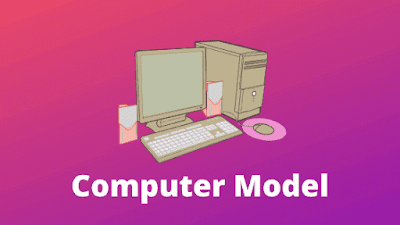Simple Model of Computer: In this tutorial, we are going to learn about the simple model of computer systems and see the main components of the computer system.## Simple Model of computer system

Mainly there are four things which can organize a computer.
1. Input
2. Memory
3. Processing Unit
4. Output### What is Input?

Input is a set of instructions that can be given to the computer to process the data.

for example, our body needs the energy to work. and to provide energy to our body we eat regular food. so in our terms, food is work as an input.
likewise, the computer needs input to work. and it can be given by mouse, keyboard, etc...

### What is Memory?

Memory is a physical space where the compute saves the data to process later. using memory we also can save the data for later and modify that data when we need it. we also can delete the data.

for example, when we eat food it comes to our stomach and stored and this stored food gives us the regular energy to work.

### What is the Processing Unit?

The processing unit is the brain of the computer system. it solves a particular problem like it preforms operations on a given problem using our instructions.

for example, if we run, walk or talk all are managed by our brain. without the brain, we are not able to work at a single time.
likewise, the processing unit is the brain of the computer and without processing, the unit computer cannot work even a single second.
like if we click on any point using the mouse then it gives the signal to the PU and processing unit react as situation.

### What is Output?

The output is the thing that comes when the computer process any information. like in the calculator if we add two numbers like 2 + 2. then result comes 4. this is the output.

for example, if we thought that you want to eat a thing then the signal goes to brain and brain work using the process. like first you pick up the thing and then eat it and this is the output.

Note: if a process does not produce any output then this process is not real.

So, these are four major components which are very important for the working of a computer system.

#### Why we need a computer Machine?

A computer machine designed to carry out algorithms for processing data and instructions. an input unit is provided to read the algorithm and the data to be processed by the algorithm. the memory unit stores the algorithm and computed value the processing unit interprets the instructions and carries them out. it has the capability to perform arithmetic operations, character manipulation operations, and logical operations. the output unit prints or display computed results.

If you like this information then please share this post as much as possible and don't forget to comment me your queries and suggestions.